# 硬币系列三 | 硬币自动分类的一个论文复现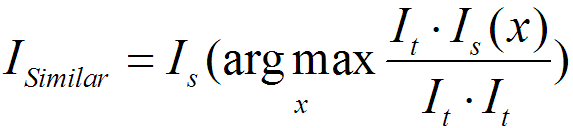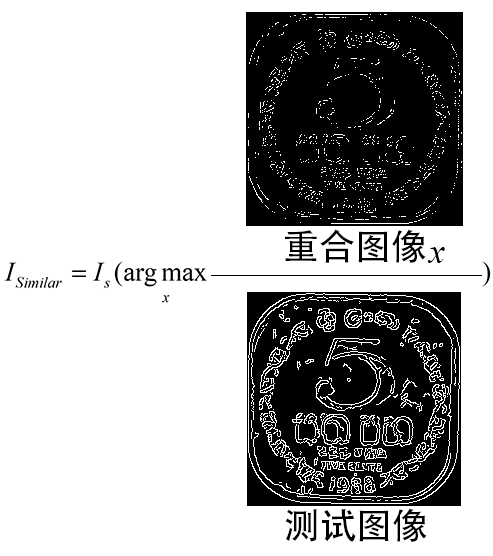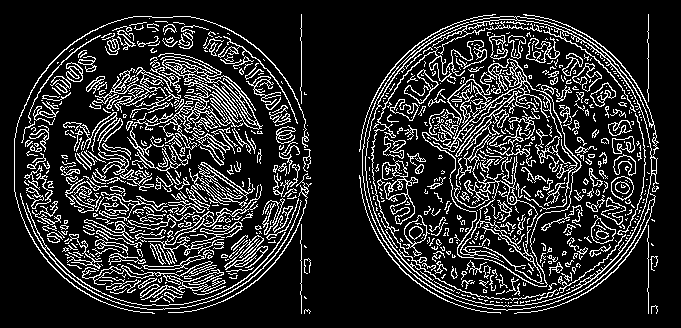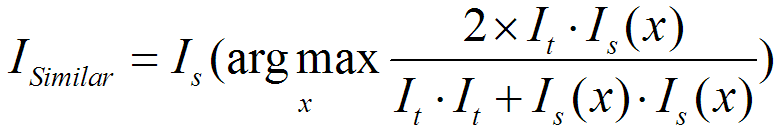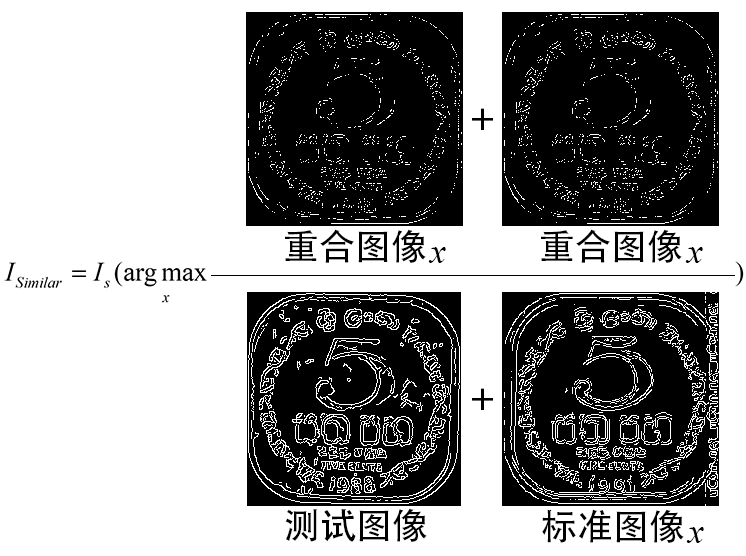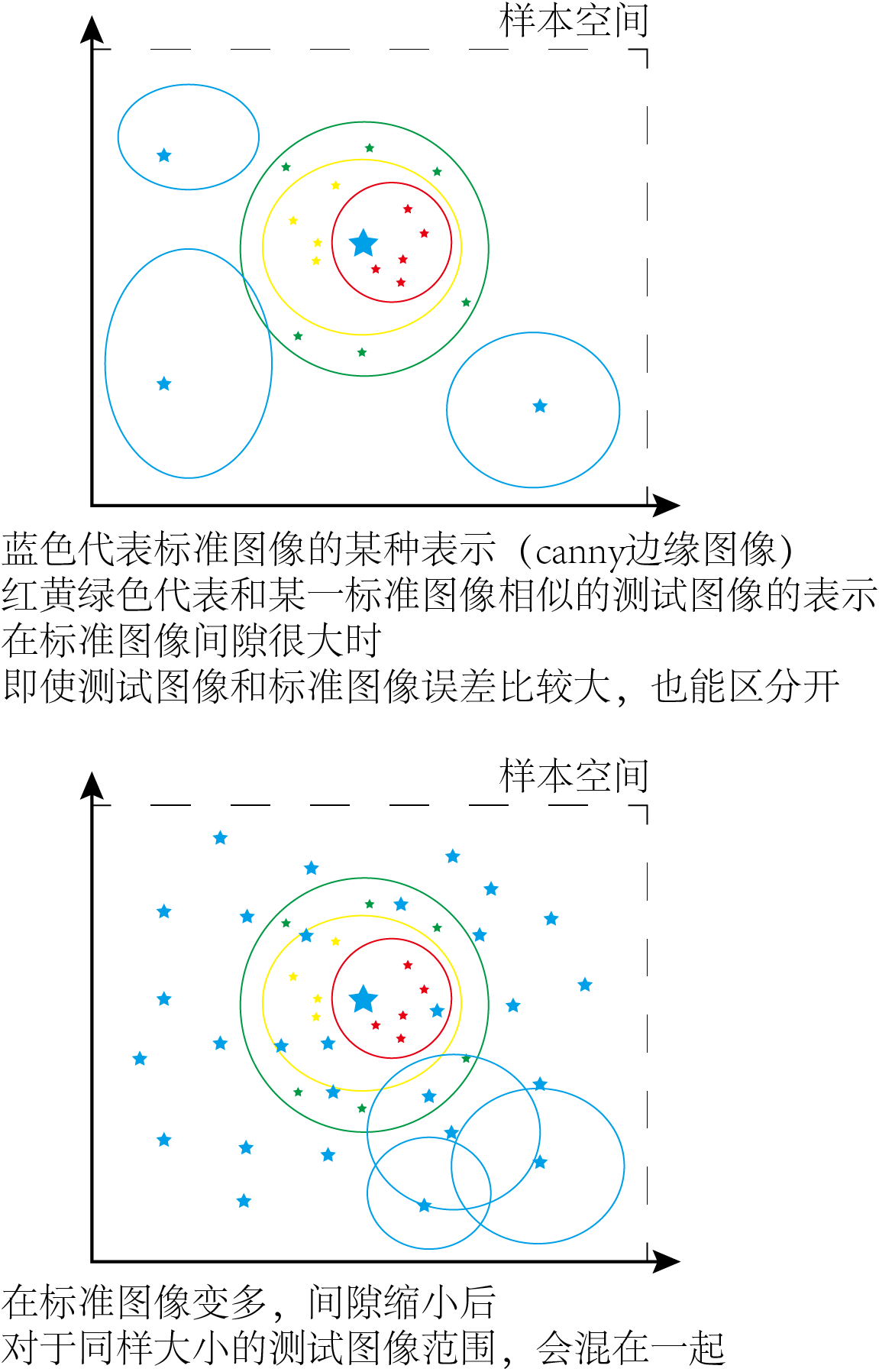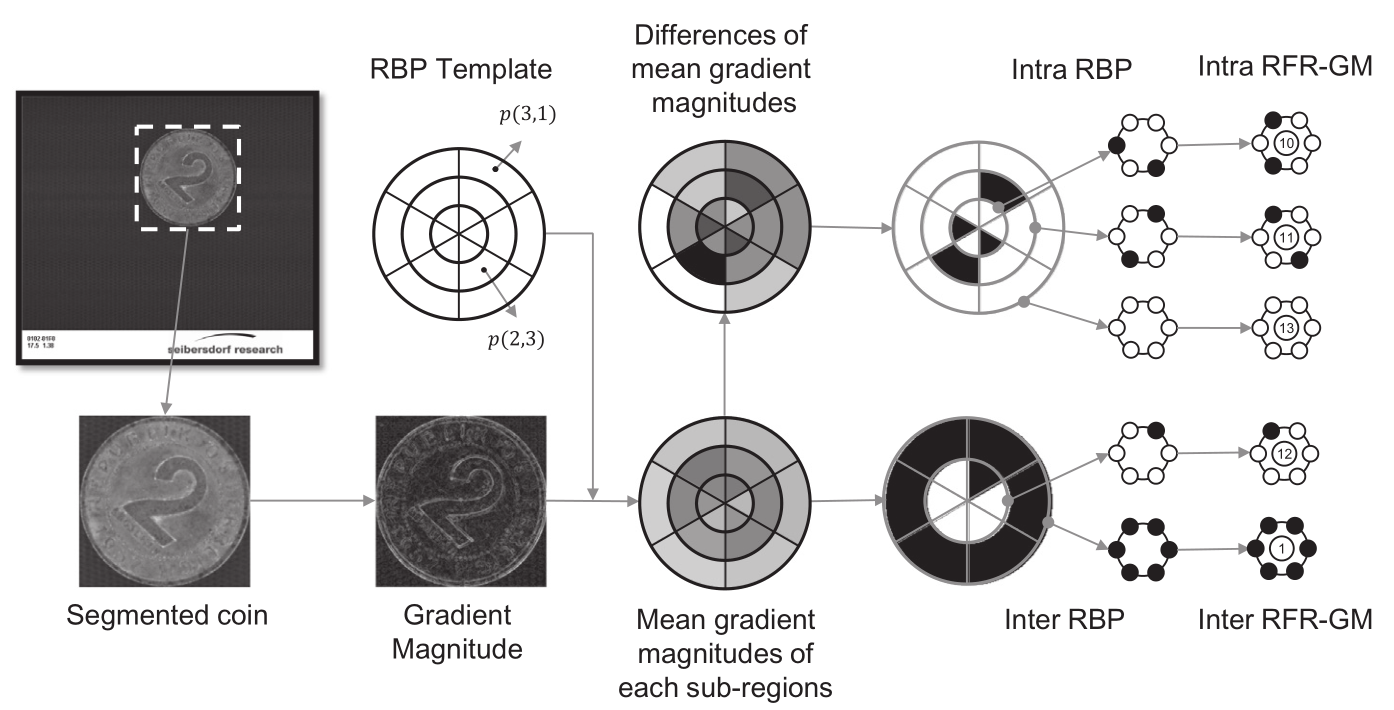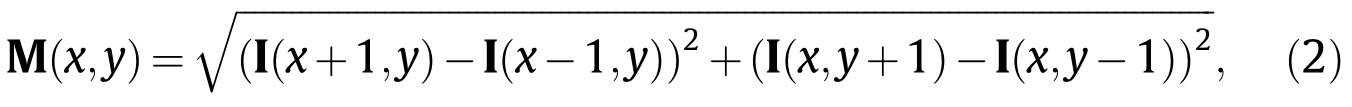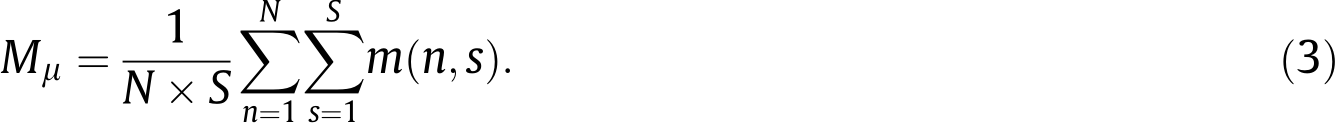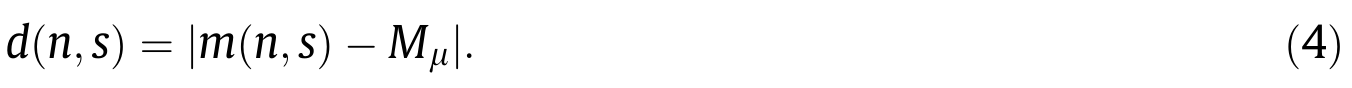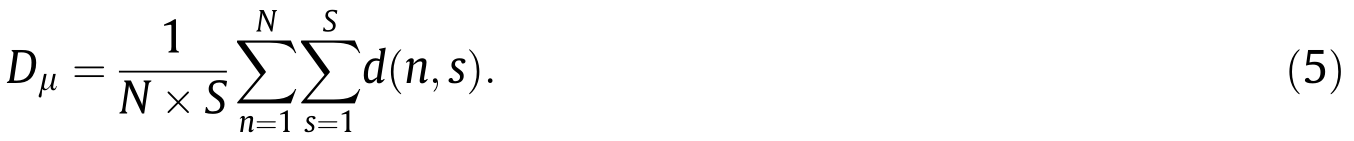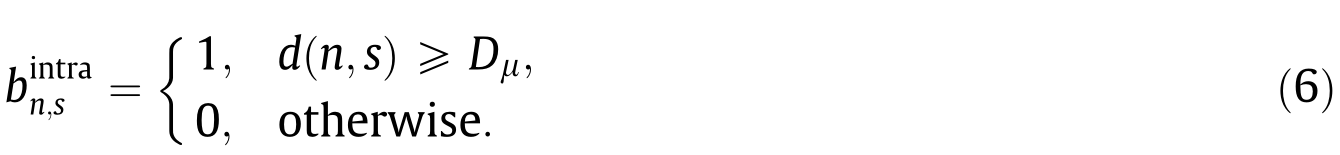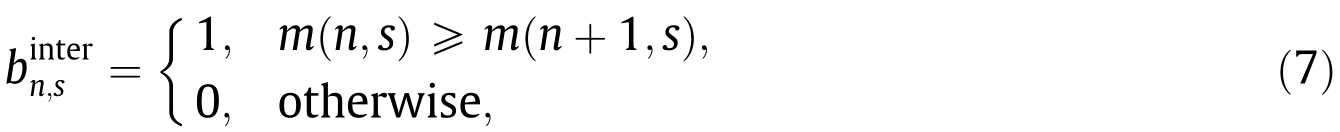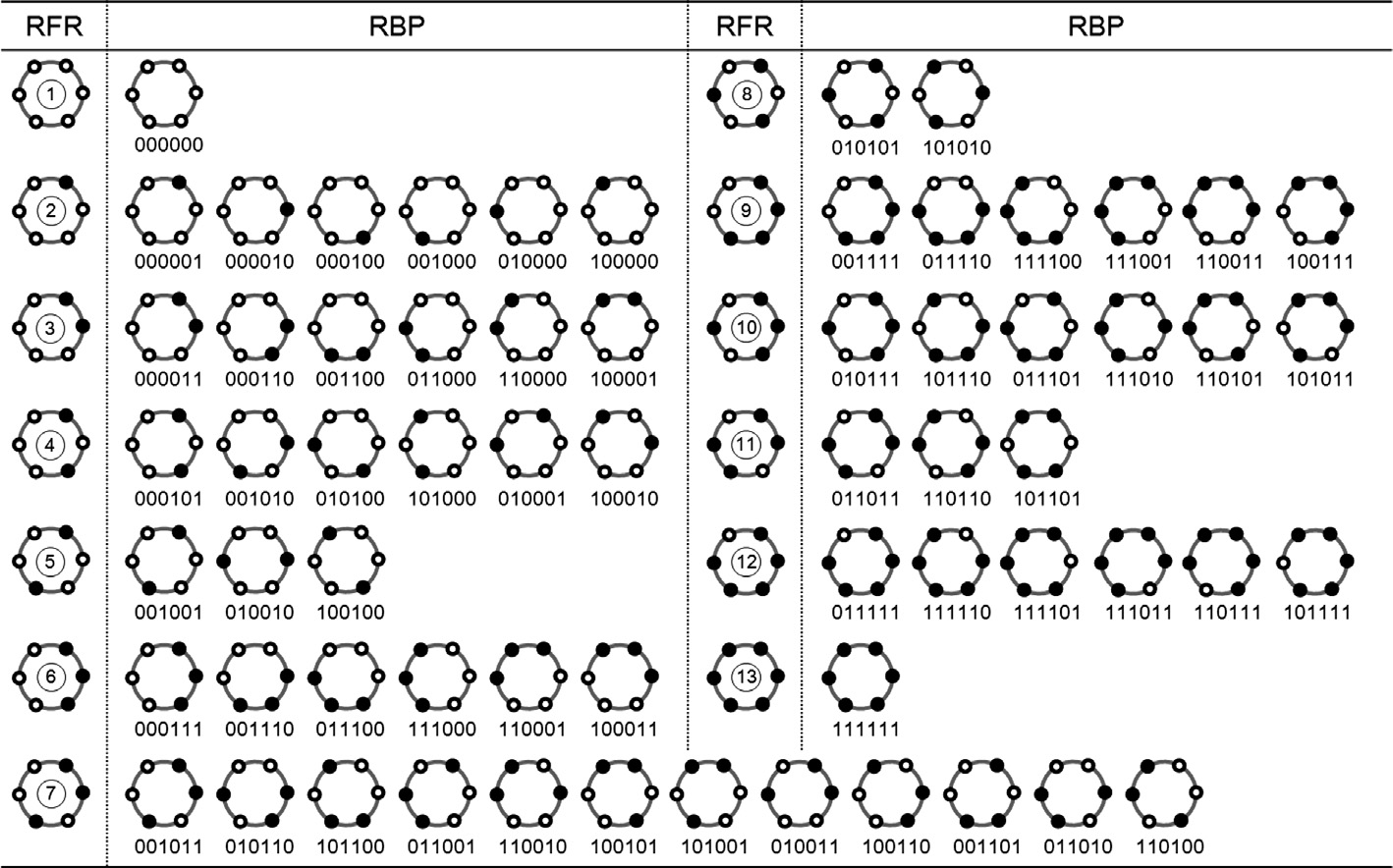M = zeros(I_size - 2);
for x = 2:I_size - 1
for y = 2:I_size - 1
M(x-1, y-1) = sqrt((I(x+1, y) - I(x-1, y))^2 + (I(x, y+1) - I(x,y-1))^2);
end
endr = (I_size - 2) / 2;
center_point = r;

axis_x = zeros(I_size - 2);
for row = 1:I_size - 2
axis_x(row,:) = [1:I_size - 2];
end

axis_y = zeros(I_size - 2);
for column = 1:I_size - 2
axis_y(:,column) = [1:I_size - 2];
end

condition_r = abs(sqrt((axis_x-center_point).^2+ (axis_y-center_point).^2));
condition_angle =atan2(axis_y-center_point, axis_x-center_point)/pi*180+180;

pic_num = 1;
m = zeros(rings, sub_regions);
for ring = 1:rings
for region = 1:sub_regions
area_r = condition_r < ring*(r/rings) & condition_r >=(ring-1)*(r/rings);
area_angle = condition_angle < region*(360/sub_regions) &condition_angle >= (region-1)*(360/sub_regions);
area_need = area_r .* area_angle;
area_gradient = M .* area_need;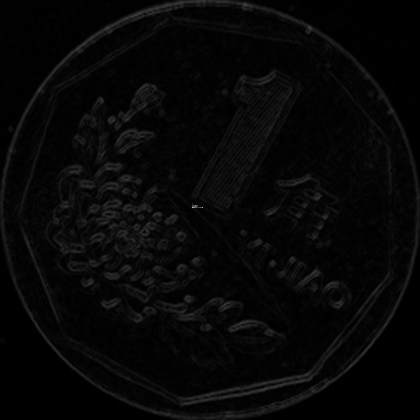%        % save a gif to observe
%        F=getframe(gcf);
%        I=frame2im(F);
%        [I,map]=rgb2ind(I,256);
%        if pic_num == 1
%            imwrite(I,map,'RFR_log/area.gif','gif','Loopcount',inf,'DelayTime',0.1);
%        else
%            imwrite(I,map,'RFR_log/area.gif','gif','WriteMode','append','DelayTime',0.1);
%        end
%        pic_num = pic_num + 1;

m(ring, region) = sum(area_gradient(:)) / sum(area_need(:));
end
end


1

IND = containers.Map;

if ~isKey(IND, dec2bin(CP_FP_dec(1),sub_regions))
for index_map = 1:length
IND(dec2bin(CP_FP_dec(index_map), sub_regions)) = ind;
end
ind = ind + 1;
end

（只记录了判断键值是否出现过，整体代码见“阅读原文”）

2

% reference:https://www.ilovematlab.cn/thread-321138-1-1.html
[m, n] = meshgrid(RFR_i_list_dec,RFR_j_list_dec');
[res(:,1), res(:,2)] = deal(reshape(m, [],1), reshape(n, [], 1));

DH = dec2bin(bitxor(res(:,1), res(:,2)));
[length, ~] = size(DH);
DH2 = uint8(zeros(length, 1));
for i = 1:length
DH2(i) = sum(DH(i,:)=='1');
end
min_Hamming = min(DH2);

key = keys(IND);
value = uint32(cell2mat(values(IND)));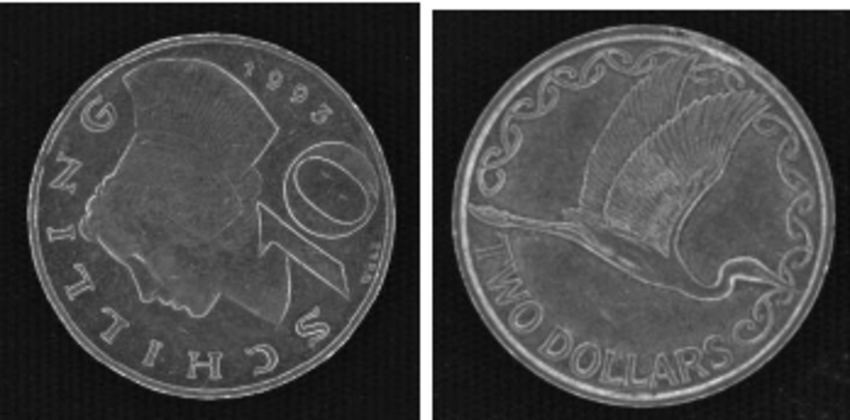https://www.frontiersin.org/articles/10.3389/fict.2017.00009/full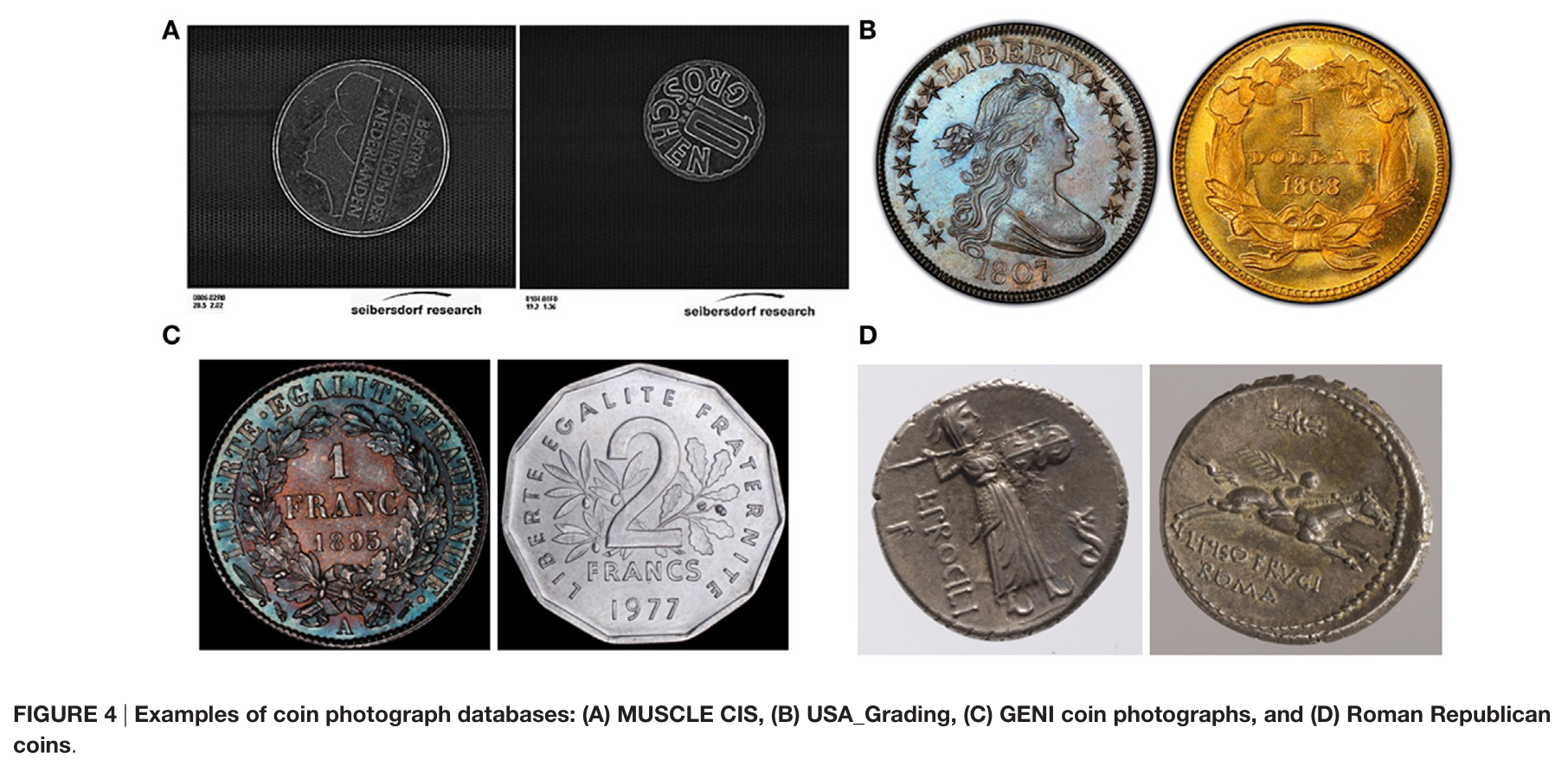（这个作者也研究过硬币分类的问题，用的貌似是类似SIFT那种方法，看起来好像很吊的样子，这篇文章的方法作为作业下节课找同学上台讲解）

 Kim S, Lee S H, Ro Y M, et al.Image-based coin recognition using rotation-invariant region binary patternsbased on gradient magnitudes[J]. Journal of Visual Communication and Image Representation, 2015: 217-223.
 Kim S, Lee S H, Ro Y M, et al. Rotationand flipping robust region binary patterns for video copy detection[J]. Journalof Visual Communication and Image Representation, 2014, 25(2): 373-383.
 Zaharieva M, Hubermoerk R, Noelle M, etal. On ancient coin classification[C]. ieee virtual reality conference, 2007:55-62.
 Pan X, Tougne L. A New Database of Digits Extracted from Coins with Hard-to-Segment Foreground for Optical CharacterRecognition Evaluation[J]. Frontiers in ICT, 2017.
 Pan X, Puritat K, Tougne L, et al. ANew Coin Segmentation and Graph-Based Identification Method for Numismatic Application[C]. international symposium on visual computing, 2014: 185-195.posted @ 2020-07-13 18:43  老潘家的潘老师  阅读(66)  评论(0编辑  收藏